## 2.4 Products of Vectors

### Learning Objectives

By the end of this section, you will be able to:

• Explain the difference between the scalar product and the vector product of two vectors.
• Determine the scalar product of two vectors.
• Determine the vector product of two vectors.
• Describe how the products of vectors are used in physics.

A vector can be multiplied by another vector but may not be divided by another vector. There are two kinds of products of vectors used broadly in physics and engineering. One kind of multiplication is a scalar multiplication of two vectors. Taking a scalar product of two vectors results in a number (a scalar), as its name indicates. Scalar products are used to define work and energy relations. For example, the work that a force (a vector) performs on an object while causing its displacement (a vector) is defined as a scalar product of the force vector with the displacement vector. A quite different kind of multiplication is a vector multiplication of vectors. Taking a vector product of two vectors returns as a result a vector, as its name suggests. Vector products are used to define other derived vector quantities. For example, in describing rotations, a vector quantity called torque is defined as a vector product of an applied force (a vector) and its distance from pivot to force (a vector). It is important to distinguish between these two kinds of vector multiplications because the scalar product is a scalar quantity and a vector product is a vector quantity.

### The Scalar Product of Two Vectors (the Dot Product)

Scalar multiplication of two vectors yields a scalar product.

### Scalar Product (Dot Product)

The scalar product $\overset{\to }{A}·\overset{\to }{B}$ of two vectors $\overset{\to }{A}$ and $\overset{\to }{B}$ is a number defined by the equation

$\overset{\to }{A}·\overset{\to }{B}=AB\,\text{cos}\,\phi ,$

where $\phi$ is the angle between the vectors (shown in (Figure)). The scalar product is also called the dot product because of the dot notation that indicates it.

In the definition of the dot product, the direction of angle $\phi$ does not matter, and $\phi$ can be measured from either of the two vectors to the other because $\text{cos}\,\phi =\text{cos}\,(\text{−}\phi )=\text{cos}\,(2\pi -\phi )$. The dot product is a negative number when $90\text{°}<\phi \le 180\text{°}$ and is a positive number when $0\text{°}\le \phi <90\text{°}$. Moreover, the dot product of two parallel vectors is $\overset{\to }{A}·\overset{\to }{B}=AB\,\text{cos}\,0\text{°}=AB$, and the dot product of two antiparallel vectors is $\overset{\to }{A}·\overset{\to }{B}=AB\,\text{cos}\,180\text{°}=\text{−}AB$. The scalar product of two orthogonal vectors vanishes: $\overset{\to }{A}·\overset{\to }{B}=AB\,\text{cos}\,90\text{°}=0$. The scalar product of a vector with itself is the square of its magnitude:

${\overset{\to }{A}}^{2}\equiv \overset{\to }{A}·\overset{\to }{A}=AA\,\text{cos}\,0\text{°}={A}^{2}.$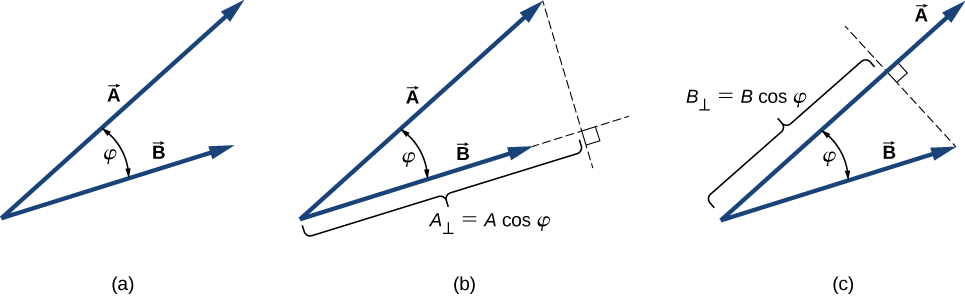Figure 2.27 The scalar product of two vectors. (a) The angle between the two vectors. (b) The orthogonal projection ${A}_{\perp }$ of vector $\overset{\to }{A}$ onto the direction of vector $\overset{\to }{B}$. (c) The orthogonal projection ${B}_{\perp }$ of vector $\overset{\to }{B}$ onto the direction of vector $\overset{\to }{A}$.

### Example

#### The Scalar Product

For the vectors shown in (Figure), find the scalar product $\overset{\to }{A}·\overset{\to }{F}$.

#### Strategy

From (Figure), the magnitudes of vectors $\overset{\to }{A}$ and $\overset{\to }{F}$ are A = 10.0 and F = 20.0. Angle $\theta$, between them, is the difference: $\theta =\phi -\alpha =110\text{°}-35\text{°}=75\text{°}$. Substituting these values into (Figure) gives the scalar product.

#### Solution

For the vectors given in (Figure), find the scalar products $\overset{\to }{A}·\overset{\to }{B}$ and $\overset{\to }{F}·\overset{\to }{C}$.

In the Cartesian coordinate system, scalar products of the unit vector of an axis with other unit vectors of axes always vanish because these unit vectors are orthogonal:

$\begin{array}{c}\hat{i}·\hat{j}=|\hat{i}||\hat{j}|\,\text{cos}\,90\text{°}=(1)(1)(0)=0,\hfill \\ \hat{i}·\hat{k}=|\hat{i}||\hat{k}|\,\text{cos}\,90\text{°}=(1)(1)(0)=0,\hfill \\ \hat{k}·\hat{j}=|\hat{k}||\hat{j}|\,\text{cos}\,90\text{°}=(1)(1)(0)=0.\hfill \end{array}$

In these equations, we use the fact that the magnitudes of all unit vectors are one: $|\hat{i}|=|\hat{j}|=|\hat{k}|=1$. For unit vectors of the axes, (Figure) gives the following identities:

$\hat{i}·\hat{i}={i}^{2}=\hat{j}·\hat{j}={j}^{2}=\hat{k}·\hat{k}={k}^{2}=1.$

The scalar product $\overset{\to }{A}·\overset{\to }{B}$ can also be interpreted as either the product of B with the orthogonal projection ${A}_{\perp }$ of vector $\overset{\to }{A}$ onto the direction of vector $\overset{\to }{B}$ ((Figure)(b)) or the product of A with the orthogonal projection ${B}_{\perp }$ of vector $\overset{\to }{B}$ onto the direction of vector $\overset{\to }{A}$ ((Figure)(c)):

$\begin{array}{ll}\hfill \overset{\to }{A}·\overset{\to }{B}& =AB\,\text{cos}\,\phi \hfill \\ & =B(A\,\text{cos}\,\phi )=B{A}_{\perp }\hfill \\ & =A(B\,\text{cos}\,\phi )=A{B}_{\perp }.\hfill \end{array}$

For example, in the rectangular coordinate system in a plane, the scalar x-component of a vector is its dot product with the unit vector $\hat{i}$, and the scalar y-component of a vector is its dot product with the unit vector $\hat{j}$:

$\{\begin{array}{l}\overset{\to }{A}·\hat{i}=|\overset{\to }{A}||\hat{i}|\,\text{cos}\,{\theta }_{A}=A\,\text{cos}\,{\theta }_{A}={A}_{x}\\ \overset{\to }{A}·\hat{j}=|\overset{\to }{A}||\hat{j}|\,\text{cos}\,(90\text{°}-{\theta }_{A})=A\,\text{sin}\,{\theta }_{A}={A}_{y}\end{array}.$

Scalar multiplication of vectors is commutative,

$\overset{\to }{A}·\overset{\to }{B}=\overset{\to }{B}·\overset{\to }{A},$

and obeys the distributive law:

$\overset{\to }{A}·(\overset{\to }{B}+\overset{\to }{C})=\overset{\to }{A}·\overset{\to }{B}+\overset{\to }{A}·\overset{\to }{C}.$

We can use the commutative and distributive laws to derive various relations for vectors, such as expressing the dot product of two vectors in terms of their scalar components.

For vector $\overset{\to }{A}={A}_{x}\hat{i}+{A}_{y}\hat{j}+{A}_{z}\hat{k}$ in a rectangular coordinate system, use (Figure) through (Figure) to show that $\overset{\to }{A}·\hat{i}={A}_{x}$ $\overset{\to }{A}·\hat{j}={A}_{y}$ and $\overset{\to }{A}·\hat{k}={A}_{z}$.

When the vectors in (Figure) are given in their vector component forms,

$\overset{\to }{A}={A}_{x}\hat{i}+{A}_{y}\hat{j}+{A}_{z}\hat{k}\,\text{and}\,\overset{\to }{B}={B}_{x}\hat{i}+{B}_{y}\hat{j}+{B}_{z}\hat{k},$

we can compute their scalar product as follows:

$\begin{array}{lll}\hfill \overset{\to }{A}·\overset{\to }{B}& =\hfill & ({A}_{x}\hat{i}+{A}_{y}\hat{j}+{A}_{z}\hat{k})·({B}_{x}\hat{i}+{B}_{y}\hat{j}+{B}_{z}\hat{k})\hfill \\ & =\hfill & \enspace{A}_{x}{B}_{x}\hat{i}·\hat{i}+{A}_{x}{B}_{y}\hat{i}·\hat{j}+{A}_{x}{B}_{z}\hat{i}·\hat{k}\hfill \\ & & +{A}_{y}{B}_{x}\hat{j}·\hat{i}+{A}_{y}{B}_{y}\hat{j}·\hat{j}+{A}_{y}{B}_{z}\hat{j}·\hat{k}\hfill \\ & & +{A}_{z}{B}_{x}\,\hat{k}·\hat{i}+{A}_{z}{B}_{y}\hat{k}·\hat{j}+{A}_{z}{B}_{z}\,\hat{k}·\hat{k}.\hfill \end{array}$

Since scalar products of two different unit vectors of axes give zero, and scalar products of unit vectors with themselves give one (see (Figure) and (Figure)), there are only three nonzero terms in this expression. Thus, the scalar product simplifies to

$\overset{\to }{A}·\overset{\to }{B}={A}_{x}{B}_{x}+{A}_{y}{B}_{y}+{A}_{z}{B}_{z}.$

We can use (Figure) for the scalar product in terms of scalar components of vectors to find the angle between two vectors. When we divide (Figure) by AB, we obtain the equation for $\text{cos}\,\phi$, into which we substitute (Figure):

$\text{cos}\,\phi =\frac{\overset{\to }{A}·\overset{\to }{B}}{AB}=\frac{{A}_{x}{B}_{x}+{A}_{y}{B}_{y}+{A}_{z}{B}_{z}}{AB}.$

Angle $\phi$ between vectors $\overset{\to }{A}$ and $\overset{\to }{B}$ is obtained by taking the inverse cosine of the expression in (Figure).

### Example

#### Angle between Two Forces

Three dogs are pulling on a stick in different directions, as shown in (Figure). The first dog pulls with force ${\overset{\to }{F}}_{1}=(10.0\hat{i}-20.4\hat{j}+2.0\hat{k})\text{N}$, the second dog pulls with force ${\overset{\to }{F}}_{2}=(-15.0\hat{i}-6.2\hat{k})\text{N}$, and the third dog pulls with force ${\overset{\to }{F}}_{3}=(5.0\hat{i}+12.5\hat{j})\text{N}$. What is the angle between forces ${\overset{\to }{F}}_{1}$ and ${\overset{\to }{F}}_{2}$?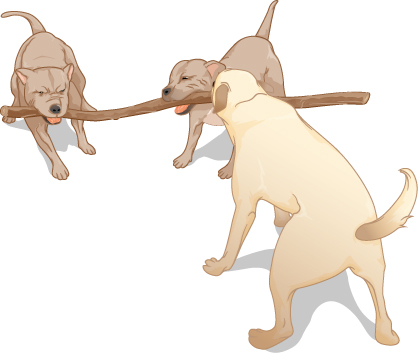Figure 2.28 Three dogs are playing with a stick.

#### Strategy

The components of force vector ${\overset{\to }{F}}_{1}$ are ${F}_{1x}=10.0\,\text{N}$, ${F}_{1y}=-20.4\,\text{N}$, and ${F}_{1z}=2.0\,\text{N}$, whereas those of force vector ${\overset{\to }{F}}_{2}$ are ${F}_{2x}=-15.0\,\text{N}$, ${F}_{2y}=0.0\,\text{N}$, and ${F}_{2z}=-6.2\,\text{N}$. Computing the scalar product of these vectors and their magnitudes, and substituting into (Figure) gives the angle of interest.

#### Significance

Notice that when vectors are given in terms of the unit vectors of axes, we can find the angle between them without knowing the specifics about the geographic directions the unit vectors represent. Here, for example, the +x-direction might be to the east and the +y-direction might be to the north. But, the angle between the forces in the problem is the same if the +x-direction is to the west and the +y-direction is to the south.

Find the angle between forces ${\overset{\to }{F}}_{1}$ and ${\overset{\to }{F}}_{3}$ in (Figure).

### Example

#### The Work of a Force

When force $\overset{\to }{F}$ pulls on an object and when it causes its displacement $\overset{\to }{D}$, we say the force performs work. The amount of work the force does is the scalar product $\overset{\to }{F}·\overset{\to }{D}$. If the stick in (Figure) moves momentarily and gets displaced by vector $\overset{\to }{D}=(-7.9\hat{j}-4.2\hat{k})\,\text{cm}$, how much work is done by the third dog in (Figure)?

#### Strategy

We compute the scalar product of displacement vector $\overset{\to }{D}$ with force vector ${\overset{\to }{F}}_{3}=(5.0\hat{i}+12.5\hat{j})\text{N}$, which is the pull from the third dog. Let’s use ${W}_{3}$ to denote the work done by force ${\overset{\to }{F}}_{3}$ on displacement $\overset{\to }{D}$.

#### Significance

The SI unit of work is called the joule $(\text{J})$, where 1 J = 1 $\text{N}·\text{m}$. The unit $\text{cm}·\text{N}$ can be written as ${10}^{-2}\text{m}·\text{N}={10}^{-2}\text{J}$, so the answer can be expressed as ${W}_{3}=-0.9875\,\text{J}\approx -1.0\,\text{J}$.

How much work is done by the first dog and by the second dog in (Figure) on the displacement in (Figure)?

### The Vector Product of Two Vectors (the Cross Product)

Vector multiplication of two vectors yields a vector product.

#### Vector Product (Cross Product)

The vector product of two vectors $\overset{\to }{A}$ and $\overset{\to }{B}$ is denoted by $\overset{\to }{A}\,×\,\overset{\to }{B}$ and is often referred to as a cross product. The vector product is a vector that has its direction perpendicular to both vectors $\overset{\to }{A}$ and $\overset{\to }{B}$. In other words, vector $\overset{\to }{A}\,×\,\overset{\to }{B}$ is perpendicular to the plane that contains vectors $\overset{\to }{A}$ and $\overset{\to }{B}$, as shown in (Figure). The magnitude of the vector product is defined as

$|\overset{\to }{A}\,×\,\overset{\to }{B}|=\,AB\,\text{sin}\,\phi ,$

where angle $\phi$, between the two vectors, is measured from vector $\overset{\to }{A}$ (first vector in the product) to vector $\overset{\to }{B}$ (second vector in the product), as indicated in (Figure), and is between $0\text{°}$ and $180\text{°}$.

According to (Figure), the vector product vanishes for pairs of vectors that are either parallel $(\phi =0\text{°})$ or antiparallel $(\phi =180\text{°})$ because $\text{sin}\,0\text{°}=\text{sin}\,180\text{°}=0$.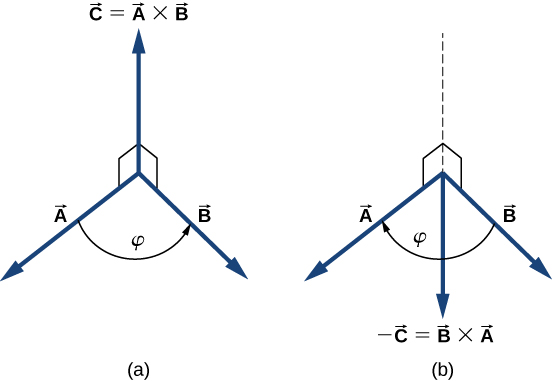Figure 2.29 The vector product of two vectors is drawn in three-dimensional space. (a) The vector product $\overset{\to }{A}\,×\,\overset{\to }{B}$ is a vector perpendicular to the plane that contains vectors $\overset{\to }{A}$ and $\overset{\to }{B}$. Small squares drawn in perspective mark right angles between $\overset{\to }{A}$ and $\overset{\to }{C}$, and between $\overset{\to }{B}$ and $\overset{\to }{C}$ so that if $\overset{\to }{A}$ and $\overset{\to }{B}$ lie on the floor, vector $\overset{\to }{C}$ points vertically upward to the ceiling. (b) The vector product $\overset{\to }{B}\,×\,\overset{\to }{A}$ is a vector antiparallel to vector $\overset{\to }{A}\,×\,\overset{\to }{B}$.

On the line perpendicular to the plane that contains vectors $\overset{\to }{A}$ and $\overset{\to }{B}$ there are two alternative directions—either up or down, as shown in (Figure)—and the direction of the vector product may be either one of them. In the standard right-handed orientation, where the angle between vectors is measured counterclockwise from the first vector, vector $\overset{\to }{A}\,×\,\overset{\to }{B}$ points upward, as seen in (Figure)(a). If we reverse the order of multiplication, so that now $\overset{\to }{B}$ comes first in the product, then vector $\overset{\to }{B}\,×\,\overset{\to }{A}$ must point downward, as seen in (Figure)(b). This means that vectors $\overset{\to }{A}\,×\,\overset{\to }{B}$ and $\overset{\to }{B}\,×\,\overset{\to }{A}$ are antiparallel to each other and that vector multiplication is not commutative but anticommutative. The anticommutative property means the vector product reverses the sign when the order of multiplication is reversed:

$\overset{\to }{A}\,×\,\overset{\to }{B}=\text{−}\overset{\to }{B}\,×\,\overset{\to }{A}.$

The corkscrew right-hand rule is a common mnemonic used to determine the direction of the vector product. As shown in (Figure), a corkscrew is placed in a direction perpendicular to the plane that contains vectors $\overset{\to }{A}$ and $\overset{\to }{B}$, and its handle is turned in the direction from the first to the second vector in the product. The direction of the cross product is given by the progression of the corkscrew.Figure 2.30 The corkscrew right-hand rule can be used to determine the direction of the cross product $\overset{\to }{A}\,×\,\overset{\to }{B}$. Place a corkscrew in the direction perpendicular to the plane that contains vectors $\overset{\to }{A}$ and $\overset{\to }{B}$, and turn it in the direction from the first to the second vector in the product. The direction of the cross product is given by the progression of the corkscrew. (a) Upward movement means the cross-product vector points up. (b) Downward movement means the cross-product vector points downward.

### Example

#### The Torque of a Force

The mechanical advantage that a familiar tool called a wrench provides ((Figure)) depends on magnitude F of the applied force, on its direction with respect to the wrench handle, and on how far from the nut this force is applied. The distance R from the nut to the point where force vector $\overset{\to }{F}$ is attached and is represented by the radial vector $\overset{\to }{R}$. The physical vector quantity that makes the nut turn is called torque (denoted by $\overset{\to }{\tau })$, and it is the vector product of the distance between the pivot to force with the force: $\overset{\to }{\tau }=\overset{\to }{R}\,×\,\overset{\to }{F}$.

To loosen a rusty nut, a 20.00-N force is applied to the wrench handle at angle $\phi =40\text{°}$ and at a distance of 0.25 m from the nut, as shown in (Figure)(a). Find the magnitude and direction of the torque applied to the nut. What would the magnitude and direction of the torque be if the force were applied at angle $\phi =45\text{°}$, as shown in (Figure)(b)? For what value of angle $\phi$ does the torque have the largest magnitude?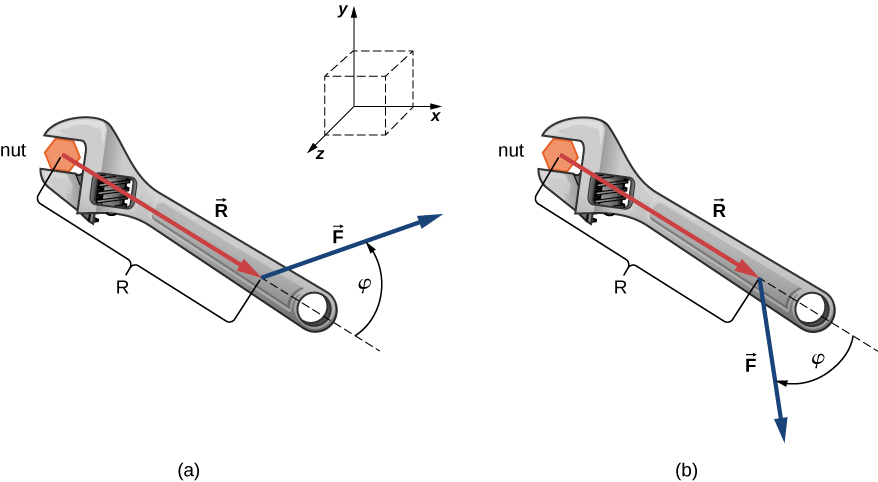Figure 2.31 A wrench provides grip and mechanical advantage in applying torque to turn a nut. (a) Turn counterclockwise to loosen the nut. (b) Turn clockwise to tighten the nut.

#### Strategy

We adopt the frame of reference shown in (Figure), where vectors $\overset{\to }{R}$ and $\overset{\to }{F}$ lie in the xy-plane and the origin is at the position of the nut. The radial direction along vector $\overset{\to }{R}$ (pointing away from the origin) is the reference direction for measuring the angle $\phi$ because $\overset{\to }{R}$ is the first vector in the vector product $\overset{\to }{\tau }=\overset{\to }{R}\,×\,\overset{\to }{F}$. Vector $\overset{\to }{\tau }$ must lie along the z-axis because this is the axis that is perpendicular to the xy-plane, where both $\overset{\to }{R}$ and $\overset{\to }{F}$ lie. To compute the magnitude $\tau$, we use (Figure). To find the direction of $\overset{\to }{\tau }$, we use the corkscrew right-hand rule ((Figure)).

#### Significance

When solving mechanics problems, we often do not need to use the corkscrew rule at all, as we’ll see now in the following equivalent solution. Notice that once we have identified that vector $\overset{\to }{R}\,×\,\overset{\to }{F}$ lies along the z-axis, we can write this vector in terms of the unit vector $\hat{k}$ of the z-axis:

$\overset{\to }{R}\,×\,\overset{\to }{F}=RF\,\text{sin}\,\phi \hat{k}.$

In this equation, the number that multiplies $\hat{k}$ is the scalar z-component of the vector $\overset{\to }{R}\,×\,\overset{\to }{F}$. In the computation of this component, care must be taken that the angle $\phi$ is measured counterclockwise from $\overset{\to }{R}$ (first vector) to $\overset{\to }{F}$ (second vector). Following this principle for the angles, we obtain $RF\,\text{sin}\,(+40\text{°})=+3.2\,\text{N}·\text{m}$ for the situation in (a), and we obtain $RF\,\text{sin}\,(-45\text{°})=-3.5\,\text{N}·\text{m}$ for the situation in (b). In the latter case, the angle is negative because the graph in (Figure) indicates the angle is measured clockwise; but, the same result is obtained when this angle is measured counterclockwise because $+(360\text{°}-45\text{°})=+315\text{°}$ and $\text{sin}\,(+315\text{°})=\text{sin}\,(-45\text{°})$. In this way, we obtain the solution without reference to the corkscrew rule. For the situation in (a), the solution is $\overset{\to }{R}\,×\,\overset{\to }{F}=+3.2\,\text{N}·\text{m}\hat{k}$; for the situation in (b), the solution is $\overset{\to }{R}\,×\,\overset{\to }{F}=-3.5\,\text{N}·\text{m}\hat{k}$.

For the vectors given in (Figure), find the vector products $\overset{\to }{A}\,×\,\overset{\to }{B}$ and $\overset{\to }{C}\,×\,\overset{\to }{F}$.

Similar to the dot product ((Figure)), the cross product has the following distributive property:

$\overset{\to }{A}\,×\,(\overset{\to }{B}+\overset{\to }{C})=\overset{\to }{A}\,×\,\overset{\to }{B}+\overset{\to }{A}\,×\,\overset{\to }{C}.$

The distributive property is applied frequently when vectors are expressed in their component forms, in terms of unit vectors of Cartesian axes.

When we apply the definition of the cross product, (Figure), to unit vectors $\hat{i}$, $\hat{j}$, and $\hat{k}$ that define the positive x-, y-, and z-directions in space, we find that

$\hat{i}\,×\,\hat{i}=\hat{j}\,×\,\hat{j}=\hat{k}\,×\,\hat{k}=0.$

All other cross products of these three unit vectors must be vectors of unit magnitudes because $\hat{i}$, $\hat{j}$, and $\hat{k}$ are orthogonal. For example, for the pair $\hat{i}$ and $\hat{j}$, the magnitude is $|\hat{i}\,×\,\hat{j}|=ij\,\text{sin}\,90\text{°}=(1)(1)(1)=1$. The direction of the vector product $\hat{i}\,×\,\hat{j}$ must be orthogonal to the xy-plane, which means it must be along the z-axis. The only unit vectors along the z-axis are $\text{−}\hat{k}$ or $+\hat{k}$. By the corkscrew rule, the direction of vector $\hat{i}\,×\,\hat{j}$ must be parallel to the positive z-axis. Therefore, the result of the multiplication $\hat{i}\,×\,\hat{j}$ is identical to $+\hat{k}$. We can repeat similar reasoning for the remaining pairs of unit vectors. The results of these multiplications are

$\{\begin{array}{l}\hat{i}\,×\,\hat{j}=+\hat{k},\\ \hat{j}\,×\,\hat{k}=+\hat{i},\\ \hat{k}\,×\,\hat{i}=+\hat{j}.\end{array}$

Notice that in (Figure), the three unit vectors $\hat{i}$, $\hat{j}$, and $\hat{k}$ appear in the cyclic order shown in a diagram in (Figure)(a). The cyclic order means that in the product formula, $\hat{i}$ follows $\hat{k}$ and comes before $\hat{j}$, or $\hat{k}$ follows $\hat{j}$ and comes before $\hat{i}$, or $\hat{j}$ follows $\hat{i}$ and comes before $\hat{k}$. The cross product of two different unit vectors is always a third unit vector. When two unit vectors in the cross product appear in the cyclic order, the result of such a multiplication is the remaining unit vector, as illustrated in (Figure)(b). When unit vectors in the cross product appear in a different order, the result is a unit vector that is antiparallel to the remaining unit vector (i.e., the result is with the minus sign, as shown by the examples in (Figure)(c) and (Figure)(d). In practice, when the task is to find cross products of vectors that are given in vector component form, this rule for the cross-multiplication of unit vectors is very useful.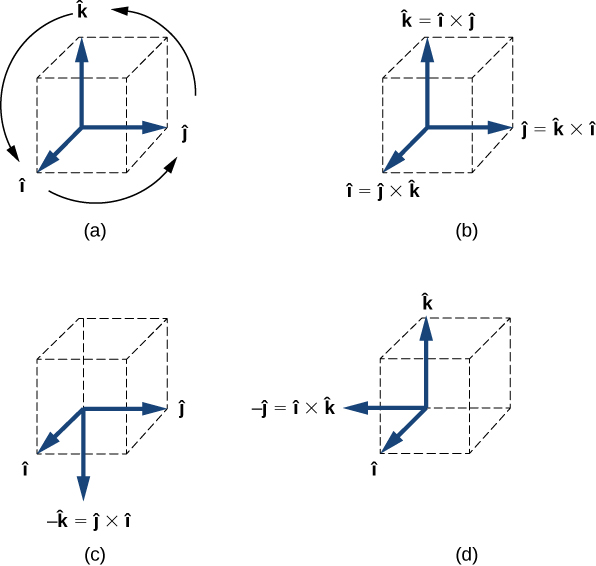Figure 2.32 (a) The diagram of the cyclic order of the unit vectors of the axes. (b) The only cross products where the unit vectors appear in the cyclic order. These products have the positive sign. (c, d) Two examples of cross products where the unit vectors do not appear in the cyclic order. These products have the negative sign.

Suppose we want to find the cross product $\overset{\to }{A}\,×\,\overset{\to }{B}$ for vectors $\overset{\to }{A}={A}_{x}\hat{i}+{A}_{y}\hat{j}+{A}_{z}\hat{k}$ and $\overset{\to }{B}={B}_{x}\hat{i}+{B}_{y}\hat{j}+{B}_{z}\hat{k}$. We can use the distributive property ((Figure)), the anticommutative property ((Figure)), and the results in (Figure) and (Figure) for unit vectors to perform the following algebra:

$\begin{array}{ccc}\hfill \overset{\to }{A}\,×\,\overset{\to }{B}& =\hfill & ({A}_{x}\hat{i}+{A}_{y}\hat{j}+{A}_{z}\hat{k})\,×\,({B}_{x}\hat{i}+{B}_{y}\hat{j}+{B}_{z}\hat{k})\hfill \\ & =\hfill & {A}_{x}\hat{i}\,×\,({B}_{x}\hat{i}+{B}_{y}\hat{j}+{B}_{z}\hat{k})+{A}_{y}\hat{j}\,×\,({B}_{x}\hat{i}+{B}_{y}\hat{j}+{B}_{z}\hat{k})+{A}_{z}\hat{k}\,×\,({B}_{x}\hat{i}+{B}_{y}\hat{j}+{B}_{z}\hat{k})\hfill \\ & =\hfill & \enspace{A}_{x}{B}_{x}\hat{i}\,×\,\hat{i}+{A}_{x}{B}_{y}\hat{i}\,×\,\hat{j}+{A}_{x}{B}_{z}\hat{i}\,×\,\hat{k}\hfill \\ & & +{A}_{y}{B}_{x}\hat{j}\,×\,\hat{i}+{A}_{y}{B}_{y}\hat{j}\,×\,\hat{j}+{A}_{y}{B}_{z}\hat{j}\,×\,\hat{k}\hfill \\ & & +{A}_{z}{B}_{x}\hat{k}\,×\,\hat{i}+{A}_{z}{B}_{y}\hat{k}\,×\,\hat{j}+{A}_{z}{B}_{z}\hat{k}\,×\,\hat{k}\hfill \\ & =\hfill & \enspace{A}_{x}{B}_{x}(0)+{A}_{x}{B}_{y}(+\hat{k})+{A}_{x}{B}_{z}(\text{−}\hat{j})\hfill \\ & & +{A}_{y}{B}_{x}(\text{−}\hat{k})+{A}_{y}{B}_{y}(0)+{A}_{y}{B}_{z}(+\hat{i})\hfill \\ & & +{A}_{z}{B}_{x}(+\hat{j})+{A}_{z}{B}_{y}(\text{−}\hat{i})+{A}_{z}{B}_{z}(0).\hfill \end{array}$

When performing algebraic operations involving the cross product, be very careful about keeping the correct order of multiplication because the cross product is anticommutative. The last two steps that we still have to do to complete our task are, first, grouping the terms that contain a common unit vector and, second, factoring. In this way we obtain the following very useful expression for the computation of the cross product:

$\overset{\to }{C}=\overset{\to }{A}\,×\,\overset{\to }{B}=({A}_{y}{B}_{z}-{A}_{z}{B}_{y})\hat{i}+({A}_{z}{B}_{x}-{A}_{x}{B}_{z})\hat{j}+({A}_{x}{B}_{y}-{A}_{y}{B}_{x})\hat{k}.$

In this expression, the scalar components of the cross-product vector are

$\{\begin{array}{c}{C}_{x}={A}_{y}{B}_{z}-{A}_{z}{B}_{y},\\ {C}_{y}={A}_{z}{B}_{x}-{A}_{x}{B}_{z},\\ {C}_{z}={A}_{x}{B}_{y}-{A}_{y}{B}_{x}.\end{array}$

When finding the cross product, in practice, we can use either (Figure) or (Figure), depending on which one of them seems to be less complex computationally. They both lead to the same final result. One way to make sure if the final result is correct is to use them both.

### Example

#### A Particle in a Magnetic Field

When moving in a magnetic field, some particles may experience a magnetic force. Without going into details—a detailed study of magnetic phenomena comes in later chapters—let’s acknowledge that the magnetic field $\overset{\to }{B}$ is a vector, the magnetic force $\overset{\to }{F}$ is a vector, and the velocity $\overset{\to }{u}$ of the particle is a vector. The magnetic force vector is proportional to the vector product of the velocity vector with the magnetic field vector, which we express as $\overset{\to }{F}=\zeta \overset{\to }{u}\,×\,\overset{\to }{B}$. In this equation, a constant $\zeta$ takes care of the consistency in physical units, so we can omit physical units on vectors $\overset{\to }{u}$ and $\overset{\to }{B}$. In this example, let’s assume the constant $\zeta$ is positive.

A particle moving in space with velocity vector $\overset{\to }{u}=-5.0\hat{i}-2.0\hat{j}+3.5\hat{k}$ enters a region with a magnetic field and experiences a magnetic force. Find the magnetic force $\overset{\to }{F}$ on this particle at the entry point to the region where the magnetic field vector is (a) $\overset{\to }{B}=7.2\hat{i}-\hat{j}-2.4\hat{k}$ and (b) $\overset{\to }{B}=4.5\hat{k}$. In each case, find magnitude F of the magnetic force and angle $\theta$ the force vector $\overset{\to }{F}$ makes with the given magnetic field vector $\overset{\to }{B}$.

#### Strategy

First, we want to find the vector product $\overset{\to }{u}\,×\,\overset{\to }{B}$, because then we can determine the magnetic force using $\overset{\to }{F}=\zeta \overset{\to }{u}\,×\,\overset{\to }{B}$. Magnitude F can be found either by using components, $F=\sqrt{{F}_{x}^{2}+{F}_{y}^{2}+{F}_{z}^{2}}$, or by computing the magnitude $|\overset{\to }{u}\,×\,\overset{\to }{B}|$ directly using (Figure). In the latter approach, we would have to find the angle between vectors $\overset{\to }{u}$ and $\overset{\to }{B}$. When we have $\overset{\to }{F}$, the general method for finding the direction angle $\theta$ involves the computation of the scalar product $\overset{\to }{F}·\overset{\to }{B}$ and substitution into (Figure). To compute the vector product we can either use (Figure) or compute the product directly, whichever way is simpler.

#### Significance

Even without actually computing the scalar product, we can predict that the magnetic force vector must always be perpendicular to the magnetic field vector because of the way this vector is constructed. Namely, the magnetic force vector is the vector product $\overset{\to }{F}=\zeta \overset{\to }{u}\,×\,\overset{\to }{B}$ and, by the definition of the vector product (see (Figure)), vector $\overset{\to }{F}$ must be perpendicular to both vectors $\overset{\to }{u}$ and $\overset{\to }{B}$.

Given two vectors $\overset{\to }{A}=\text{−}\hat{i}+\hat{j}$ and $\overset{\to }{B}=3\hat{i}-\hat{j}$, find (a) $\overset{\to }{A}\,×\,\overset{\to }{B}$, (b) $|\overset{\to }{A}\,×\,\overset{\to }{B}|$, (c) the angle between $\overset{\to }{A}$ and $\overset{\to }{B}$, and (d) the angle between $\overset{\to }{A}\,×\,\overset{\to }{B}$ and vector $\overset{\to }{C}=\hat{i}+\hat{k}$.

In conclusion to this section, we want to stress that “dot product” and “cross product” are entirely different mathematical objects that have different meanings. The dot product is a scalar; the cross product is a vector. Later chapters use the terms dot product and scalar product interchangeably. Similarly, the terms cross product and vector product are used interchangeably.

### Summary

• There are two kinds of multiplication for vectors. One kind of multiplication is the scalar product, also known as the dot product. The other kind of multiplication is the vector product, also known as the cross product. The scalar product of vectors is a number (scalar). The vector product of vectors is a vector.
• Both kinds of multiplication have the distributive property, but only the scalar product has the commutative property. The vector product has the anticommutative property, which means that when we change the order in which two vectors are multiplied, the result acquires a minus sign.
• The scalar product of two vectors is obtained by multiplying their magnitudes with the cosine of the angle between them. The scalar product of orthogonal vectors vanishes; the scalar product of antiparallel vectors is negative.
• The vector product of two vectors is a vector perpendicular to both of them. Its magnitude is obtained by multiplying their magnitudes by the sine of the angle between them. The direction of the vector product can be determined by the corkscrew right-hand rule. The vector product of two either parallel or antiparallel vectors vanishes. The magnitude of the vector product is largest for orthogonal vectors.
• The scalar product of vectors is used to find angles between vectors and in the definitions of derived scalar physical quantities such as work or energy.
• The cross product of vectors is used in definitions of derived vector physical quantities such as torque or magnetic force, and in describing rotations.

### Key Equations

 Multiplication by a scalar (vector equation) $\overset{\to }{B}=\alpha \overset{\to }{A}$ Multiplication by a scalar (scalar equation for magnitudes) $B=|\alpha |A$ Resultant of two vectors ${\overset{\to }{D}}_{AD}={\overset{\to }{D}}_{AC}+{\overset{\to }{D}}_{CD}$ Commutative law $\overset{\to }{A}+\overset{\to }{B}=\overset{\to }{B}+\overset{\to }{A}$ Associative law $(\overset{\to }{A}+\overset{\to }{B})+\overset{\to }{C}=\overset{\to }{A}+(\overset{\to }{B}+\overset{\to }{C})$ Distributive law ${\alpha }_{1}\overset{\to }{A}+{\alpha }_{2}\overset{\to }{A}=({\alpha }_{1}+{\alpha }_{2})\overset{\to }{A}$ The component form of a vector in two dimensions $\overset{\to }{A}={A}_{x}\hat{i}+{A}_{y}\hat{j}$ Scalar components of a vector in two dimensions $\{\begin{array}{c}{A}_{x}={x}_{e}-{x}_{b}\hfill \\ {A}_{y}={y}_{e}-{y}_{b}\hfill \end{array}$ Magnitude of a vector in a plane $A=\sqrt{{A}_{x}^{2}+{A}_{y}^{2}}$ The direction angle of a vector in a plane ${\theta }_{A}={\text{tan}}^{-1}(\frac{{A}_{y}}{{A}_{x}})$ Scalar components of a vector in a plane $\{\begin{array}{c}{A}_{x}=A\,\text{cos}\,{\theta }_{A}\hfill \\ {A}_{y}=A\,\text{sin}\,{\theta }_{A}\hfill \end{array}$ Polar coordinates in a plane $\{\begin{array}{c}x=r\,\text{cos}\,\phi \hfill \\ y=r\,\text{sin}\,\phi \hfill \end{array}$ The component form of a vector in three dimensions $\overset{\to }{A}={A}_{x}\hat{i}+{A}_{y}\hat{j}+{A}_{z}\hat{k}$ The scalar z-component of a vector in three dimensions ${A}_{z}={z}_{e}-{z}_{b}$ Magnitude of a vector in three dimensions $A=\sqrt{{A}_{x}^{2}+{A}_{y}^{2}+{A}_{z}^{2}}$ Distributive property $\alpha (\overset{\to }{A}+\overset{\to }{B})=\alpha \overset{\to }{A}+\alpha \overset{\to }{B}$ Antiparallel vector to $\overset{\to }{A}$ $\text{−}\overset{\to }{A}=\text{−}{A}_{x}\hat{i}-{A}_{y}\hat{j}-{A}_{z}\hat{k}$ Equal vectors $\overset{\to }{A}=\overset{\to }{B}\enspace⇔\enspace\{\begin{array}{c}{A}_{x}={B}_{x}\hfill \\ {A}_{y}={B}_{y}\hfill \\ {A}_{z}={B}_{z}\hfill \end{array}$ Components of the resultant of N vectors $\{\begin{array}{c}{F}_{Rx}=\sum _{k=1}^{N}{F}_{kx}={F}_{1x}+{F}_{2x}+\text{…}+{F}_{Nx}\hfill \\ {F}_{Ry}=\sum _{k=1}^{N}{F}_{ky}={F}_{1y}+{F}_{2y}+\text{…}+{F}_{Ny}\hfill \\ {F}_{Rz}=\sum _{k=1}^{N}{F}_{kz}={F}_{1z}+{F}_{2z}+\text{…}+{F}_{Nz}\hfill \end{array}$ General unit vector $\hat{V}=\frac{\overset{\to }{V}}{V}$ Definition of the scalar product $\overset{\to }{A}·\overset{\to }{B}=AB\,\text{cos}\,\phi$ Commutative property of the scalar product $\overset{\to }{A}·\overset{\to }{B}=\overset{\to }{B}·\overset{\to }{A}$ Distributive property of the scalar product $\overset{\to }{A}·(\overset{\to }{B}+\overset{\to }{C})=\overset{\to }{A}·\overset{\to }{B}+\overset{\to }{A}·\overset{\to }{C}$ Scalar product in terms of scalar components of vectors $\overset{\to }{A}·\overset{\to }{B}={A}_{x}{B}_{x}+{A}_{y}{B}_{y}+{A}_{z}{B}_{z}$ Cosine of the angle between two vectors $\text{cos}\,\phi =\frac{\overset{\to }{A}·\overset{\to }{B}}{AB}$ Dot products of unit vectors $\hat{i}·\hat{j}=\hat{j}·\hat{k}=\hat{k}·\hat{i}=0$ Magnitude of the vector product (definition) $|\overset{\to }{A}\,×\,\overset{\to }{B}|=AB\,\text{sin}\,\phi$ Anticommutative property of the vector product $\overset{\to }{A}\,×\,\overset{\to }{B}=\text{−}\overset{\to }{B}\,×\,\overset{\to }{A}$ Distributive property of the vector product $\overset{\to }{A}\,×\,(\overset{\to }{B}+\overset{\to }{C})=\overset{\to }{A}\,×\,\overset{\to }{B}+\overset{\to }{A}\,×\,\overset{\to }{C}$ Cross products of unit vectors $\{\begin{array}{l}\hat{i}\,×\,\hat{j}=+\hat{k},\hfill \\ \hat{j}\,×\,\hat{k}=+\hat{i},\hfill \\ \hat{k}\,×\,\hat{i}=+\hat{j}.\hfill \end{array}$ The cross product in terms of scalar components of vectors $\overset{\to }{A}\,×\,\overset{\to }{B}=({A}_{y}{B}_{z}-{A}_{z}{B}_{y})\hat{i}+({A}_{z}{B}_{x}-{A}_{x}{B}_{z})\hat{j}+({A}_{x}{B}_{y}-{A}_{y}{B}_{x})\hat{k}$

### Conceptual Questions

What is wrong with the following expressions? How can you correct them? (a) $C=\overset{\to }{A}\overset{\to }{B}$, (b) $\overset{\to }{C}=\overset{\to }{A}\overset{\to }{B}$, (c) $C=\overset{\to }{A}\,×\,\overset{\to }{B}$, (d) $C=A\overset{\to }{B}$, (e) $C+2\overset{\to }{A}=B$, (f) $\overset{\to }{C}=A\,×\,\overset{\to }{B}$, (g) $\overset{\to }{A}·\overset{\to }{B}=\overset{\to }{A}\,×\,\overset{\to }{B}$, (h) $\overset{\to }{C}=2\overset{\to }{A}·\overset{\to }{B}$, (i) $C=\overset{\to }{A}\text{/}\overset{\to }{B}$, and (j) $C=\overset{\to }{A}\text{/}B$.

If the cross product of two vectors vanishes, what can you say about their directions?

If the dot product of two vectors vanishes, what can you say about their directions?

What is the dot product of a vector with the cross product that this vector has with another vector?

### Problems

Assuming the +x-axis is horizontal to the right for the vectors in the following figure, find the following scalar products: (a) $\overset{\to }{A}·\overset{\to }{C}$, (b) $\overset{\to }{A}·\overset{\to }{F}$, (c) $\overset{\to }{D}·\overset{\to }{C}$, (d) $\overset{\to }{A}·(\overset{\to }{F}+2\overset{\to }{C})$, (e) $\hat{i}·\overset{\to }{B}$, (f) $\hat{j}·\overset{\to }{B}$, (g) $(3\hat{i}-\hat{j})·\overset{\to }{B}$, and (h) $\hat{B}·\overset{\to }{B}$.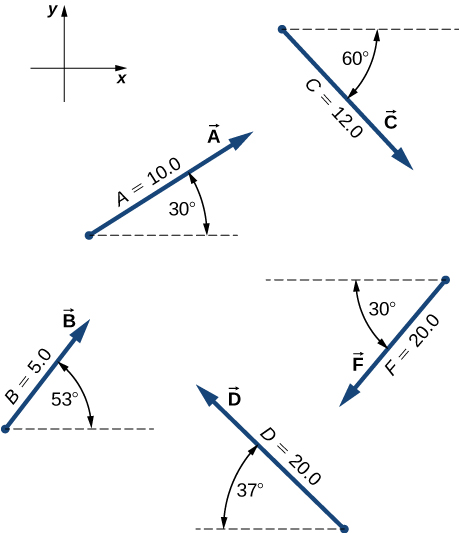Assuming the +x-axis is horizontal to the right for the vectors in the preceding figure, find (a) the component of vector $\overset{\to }{A}$ along vector $\overset{\to }{C}$, (b) the component of vector $\overset{\to }{C}$ along vector $\overset{\to }{A}$, (c) the component of vector $\hat{i}$ along vector $\overset{\to }{F}$, and (d) the component of vector $\overset{\to }{F}$ along vector $\hat{i}$.

Find the angle between vectors for (a) $\overset{\to }{D}=(-3.0\hat{i}-4.0\hat{j})\text{m}$ and $\overset{\to }{A}=(-3.0\hat{i}+4.0\hat{j})\text{m}$ and (b) $\overset{\to }{D}=(2.0\hat{i}-4.0\hat{j}+\hat{k})\text{m}$ and $\overset{\to }{B}=(-2.0\hat{i}+3.0\hat{j}+2.0\hat{k})\text{m}$.

Find the angles that vector $\overset{\to }{D}=(2.0\hat{i}-4.0\hat{j}+\hat{k})\text{m}$ makes with the x-, y-, and z– axes.

Show that the force vector $\overset{\to }{D}=(2.0\hat{i}-4.0\hat{j}+\hat{k})\text{N}$ is orthogonal to the force vector $\overset{\to }{G}=(3.0\hat{i}+4.0\hat{j}+10.0\hat{k})\text{N}$.

Assuming the +x-axis is horizontal to the right for the vectors in the previous figure, find the following vector products: (a) $\overset{\to }{A}\,×\,\overset{\to }{C}$, (b) $\overset{\to }{A}\,×\,\overset{\to }{F}$, (c) $\overset{\to }{D}\,×\,\overset{\to }{C}$, (d) $\overset{\to }{A}\,×\,(\overset{\to }{F}+2\overset{\to }{C})$, (e) $\hat{i}\,×\,\overset{\to }{B}$, (f) $\hat{j}\,×\,\overset{\to }{B}$, (g) $(3\hat{i}-\hat{j})\,×\,\overset{\to }{B}$, and (h) $\hat{B}\,×\,\overset{\to }{B}$.

Find the cross product $\overset{\to }{A}\,×\,\overset{\to }{C}$ for (a) $\overset{\to }{A}=2.0\hat{i}-4.0\hat{j}+\hat{k}$ and $\overset{\to }{C}=3.0\hat{i}+4.0\hat{j}+10.0\hat{k}$, (b) $\overset{\to }{A}=3.0\hat{i}+4.0\hat{j}+10.0\hat{k}$ and $\overset{\to }{C}=2.0\hat{i}-4.0\hat{j}+\hat{k}$, (c) $\overset{\to }{A}=-3.0\hat{i}-4.0\hat{j}$ and $\overset{\to }{C}=-3.0\hat{i}+4.0\hat{j}$, and (d) $\overset{\to }{C}=-2.0\hat{i}+3.0\hat{j}+2.0\hat{k}$ and $\overset{\to }{A}=-9.0\hat{j}$.

For the vectors in the earlier figure, find (a) $(\overset{\to }{A}\,×\,\overset{\to }{F})·\overset{\to }{D}$, (b) $(\overset{\to }{A}\,×\,\overset{\to }{F})·(\overset{\to }{D}\,×\,\overset{\to }{B})$, and (c) $(\overset{\to }{A}·\overset{\to }{F})(\overset{\to }{D}\,×\,\overset{\to }{B})$.

(a) If $\overset{\to }{A}\,×\,\overset{\to }{F}=\overset{\to }{B}\,×\,\overset{\to }{F}$, can we conclude $\overset{\to }{A}=\overset{\to }{B}$? (b) If $\overset{\to }{A}·\overset{\to }{F}=\overset{\to }{B}·\overset{\to }{F}$, can we conclude $\overset{\to }{A}=\overset{\to }{B}$? (c) If $F\overset{\to }{A}=\overset{\to }{B}F$, can we conclude $\overset{\to }{A}=\overset{\to }{B}$? Why or why not?

You fly $32.0\,\text{km}$ in a straight line in still air in the direction $35.0\text{°}$ south of west. (a) Find the distances you would have to fly due south and then due west to arrive at the same point. (b) Find the distances you would have to fly first in a direction $45.0\text{°}$ south of west and then in a direction $45.0\text{°}$ west of north. Note these are the components of the displacement along a different set of axes—namely, the one rotated by $45\text{°}$ with respect to the axes in (a).

Rectangular coordinates of a point are given by (2, y) and its polar coordinates are given by $(r,\pi \text{/}6)$. Find y and r.

If the polar coordinates of a point are $(r,\phi )$ and its rectangular coordinates are $(x,y)$, determine the polar coordinates of the following points: (a) (−x, y), (b) (−2x, −2y), and (c) (3x, −3y).

Vectors $\overset{\to }{A}$ and $\overset{\to }{B}$ have identical magnitudes of 5.0 units. Find the angle between them if $\overset{\to }{A}+\overset{\to }{B}=5\sqrt{2}\hat{j}$.

Starting at the island of Moi in an unknown archipelago, a fishing boat makes a round trip with two stops at the islands of Noi and Poi. It sails from Moi for 4.76 nautical miles (nmi) in a direction $37\text{°}$ north of east to Noi. From Noi, it sails $69\text{°}$ west of north to Poi. On its return leg from Poi, it sails $28\text{°}$ east of south. What distance does the boat sail between Noi and Poi? What distance does it sail between Moi and Poi? Express your answer both in nautical miles and in kilometers. Note: 1 nmi = 1852 m.

An air traffic controller notices two signals from two planes on the radar monitor. One plane is at altitude 800 m and in a 19.2-km horizontal distance to the tower in a direction $25\text{°}$ south of west. The second plane is at altitude 1100 m and its horizontal distance is 17.6 km and $20\text{°}$ south of west. What is the distance between these planes?

Show that when $\overset{\to }{A}+\overset{\to }{B}=\overset{\to }{C}$, then ${C}^{2}={A}^{2}+{B}^{2}+2AB\,\text{cos}\,\phi$, where $\phi$ is the angle between vectors $\overset{\to }{A}$ and $\overset{\to }{B}$.

Four force vectors each have the same magnitude f. What is the largest magnitude the resultant force vector may have when these forces are added? What is the smallest magnitude of the resultant? Make a graph of both situations.

A skater glides along a circular path of radius 5.00 m in clockwise direction. When he coasts around one-half of the circle, starting from the west point, find (a) the magnitude of his displacement vector and (b) how far he actually skated. (c) What is the magnitude of his displacement vector when he skates all the way around the circle and comes back to the west point?

A stubborn dog is being walked on a leash by its owner. At one point, the dog encounters an interesting scent at some spot on the ground and wants to explore it in detail, but the owner gets impatient and pulls on the leash with force $\overset{\to }{F}=(98.0\hat{i}+132.0\hat{j}+32.0\hat{k})\text{N}$ along the leash. (a) What is the magnitude of the pulling force? (b) What angle does the leash make with the vertical?

If the velocity vector of a polar bear is $\overset{\to }{u}=(-18.0\hat{i}-13.0\hat{j})\text{km}\text{/}\text{h}$, how fast and in what geographic direction is it heading? Here, $\hat{i}$ and $\hat{j}$ are directions to geographic east and north, respectively.

Find the scalar components of three-dimensional vectors $\overset{\to }{G}$ and $\overset{\to }{H}$ in the following figure and write the vectors in vector component form in terms of the unit vectors of the axes.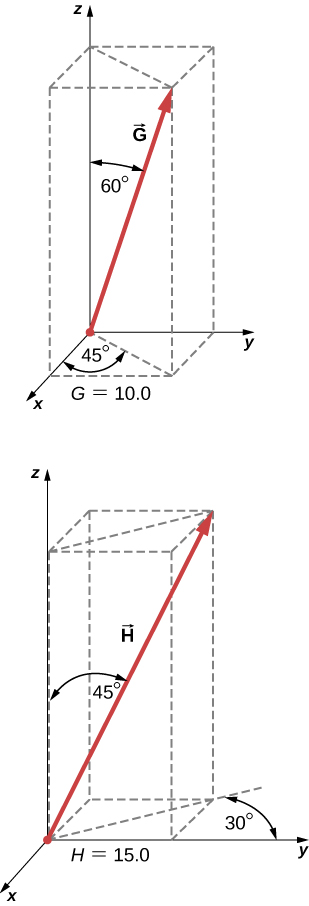A diver explores a shallow reef off the coast of Belize. She initially swims 90.0 m north, makes a turn to the east and continues for 200.0 m, then follows a big grouper for 80.0 m in the direction $30\text{°}$ north of east. In the meantime, a local current displaces her by 150.0 m south. Assuming the current is no longer present, in what direction and how far should she now swim to come back to the point where she started?

A force vector $\overset{\to }{A}$ has x– and y-components, respectively, of −8.80 units of force and 15.00 units of force. The x– and y-components of force vector $\overset{\to }{B}$ are, respectively, 13.20 units of force and −6.60 units of force. Find the components of force vector $\overset{\to }{C}$ that satisfies the vector equation $\overset{\to }{A}-\overset{\to }{B}+3\overset{\to }{C}=0$.

Vectors $\overset{\to }{A}$ and $\overset{\to }{B}$ are two orthogonal vectors in the xy-plane and they have identical magnitudes. If $\overset{\to }{A}=3.0\hat{i}+4.0\hat{j}$, find $\overset{\to }{B}$.

For the three-dimensional vectors in the following figure, find (a) $\overset{\to }{G}\,×\,\overset{\to }{H}$, (b) $|\overset{\to }{G}\,×\,\overset{\to }{H}|$, and (c) $\overset{\to }{G}·\overset{\to }{H}$.Show that $(\overset{\to }{B}\,×\,\overset{\to }{C})·\overset{\to }{A}$ is the volume of the parallelepiped, with edges formed by the three vectors in the following figure.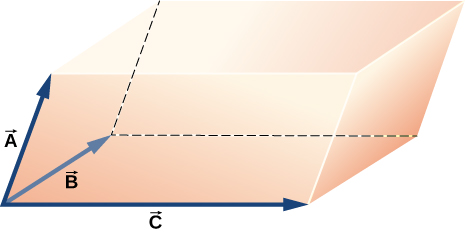proof

### Challenge Problems

Vector $\overset{\to }{B}$ is 5.0 cm long and vector $\overset{\to }{A}$ is 4.0 cm long. Find the angle between these two vectors when $|\overset{\to }{A}+\overset{\to }{B}|=\,3.0\,\text{cm}$ and $|\overset{\to }{A}-\overset{\to }{B}|=\,3.0\,\text{cm}$.

What is the component of the force vector $\overset{\to }{G}=(3.0\hat{i}+4.0\hat{j}+10.0\hat{k})\text{N}$ along the force vector $\overset{\to }{H}=(1.0\hat{i}+4.0\hat{j})\text{N}$?

The following figure shows a triangle formed by the three vectors $\overset{\to }{A}$, $\overset{\to }{B}$, and $\overset{\to }{C}$. If vector ${\overset{\to }{C}}^{\prime }$ is drawn between the midpoints of vectors $\overset{\to }{A}$ and $\overset{\to }{B}$, show that ${\overset{\to }{C}}^{\prime }=\overset{\to }{C}\text{/}2$.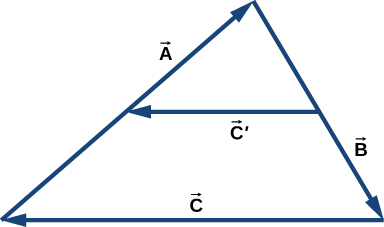Distances between points in a plane do not change when a coordinate system is rotated. In other words, the magnitude of a vector is invariant under rotations of the coordinate system. Suppose a coordinate system S is rotated about its origin by angle $\phi$ to become a new coordinate system ${\text{S}}^{\prime }$, as shown in the following figure. A point in a plane has coordinates (x, y) in S and coordinates $({x}^{\prime },{y}^{\prime })$ in ${\text{S}}^{\prime }$.

(a) Show that, during the transformation of rotation, the coordinates in ${\text{S}}^{\prime }$ are expressed in terms of the coordinates in S by the following relations:

$\{\begin{array}{c}{x}^{\prime }=x\,\text{cos}\,\phi +y\,\text{sin}\,\phi \\ {y}^{\prime }=\text{−}x\,\text{sin}\,\phi +y\,\text{cos}\,\phi \end{array}.$

(b) Show that the distance of point P to the origin is invariant under rotations of the coordinate system. Here, you have to show that

$\sqrt{{x}^{2}+{y}^{2}}=\sqrt{{{x}^{\prime }}^{2}+{{y}^{\prime }}^{2}}.$

(c) Show that the distance between points P and Q is invariant under rotations of the coordinate system. Here, you have to show that

$\sqrt{{({x}_{P}-{x}_{Q})}^{2}+{({y}_{P}-{y}_{Q})}^{2}}=\sqrt{{({{x}^{\prime }}_{P}-{{x}^{\prime }}_{Q})}^{2}+{({{y}^{\prime }}_{P}-{{y}^{\prime }}_{Q})}^{2}}.$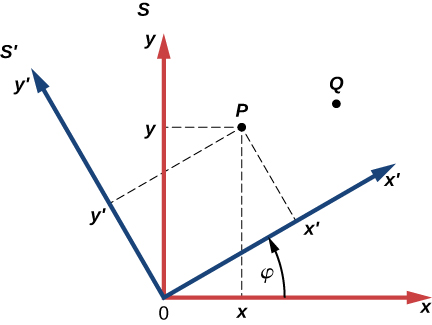proof

### Glossary

anticommutative property
change in the order of operation introduces the minus sign
corkscrew right-hand rule
a rule used to determine the direction of the vector product
cross product
the result of the vector multiplication of vectors is a vector called a cross product; also called a vector product
dot product
the result of the scalar multiplication of two vectors is a scalar called a dot product; also called a scalar product
scalar product
the result of the scalar multiplication of two vectors is a scalar called a scalar product; also called a dot product
vector product
the result of the vector multiplication of vectors is a vector called a vector product; also called a cross product